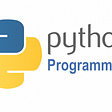# Type Inference by Example, Part 3

Continuing where we left off in part 2, we’ll now consider calling a generic function:

`function map<A, B>(array : Array<A>, body : A => B) : Array<B>`

So this is the classic `map` function that applies a lambda function `body` to each element of the `array` and returns a new array with the results. Note that `map` takes two type parameters, `A` and `B`.

Here’s a function that squares each element of an array:

`function square(items) {    return map(items, x => x * x);}`

Now, to add in the missing type annotations, we first must instantiate the type for `map` — that is, create a new copy of the function signature where every type parameter is replaced with a fresh type variable:

`function map<\$3, \$4>(array : Array<\$3>, body : \$3 => \$4) : Array<\$4>`

While adding the missing type annotations, we make the generics explicit at the call site of `map`:

`function square(items : \$1) : \$2 {    return map<\$3, \$4>(items, (x : \$5) => x * x);}`

The first parameter of our instantiated `map` has type `Array<\$3>`, so we get the following constraint:

`\$1 == Array<\$3>`

Considering the lambda function, and assuming that `* : (Int, Int) => Int`, we get the duplicate constraint:

`\$5 == Int\$5 == Int`

And from the return type of `*` we know that the return type of the lambda must be `Int`, such that the full type of the lambda becomes `\$5 => Int`. It should be the same type as the second parameter to `map`, so we get the constraint:

`(\$5 => Int) == (\$3 => \$4)`

From the `return` statement, we know that the return type of `square` is the same as the return type of `map`:

`\$2 == Array<\$4>`

Now we have all the constraints:

`\$1 == Array<\$3>\$5 == Int\$5 == Int(\$5 => Int) == (\$3 => \$4)\$2 == Array<\$4>`

Since `(\$5 => Int) == (\$3 => \$4)` has the same type constructor `=>` on both sides, we can replace it by two simpler constraints `\$5 == \$3` and `Int == \$4`:

`\$1 == Array<\$3>\$5 == Int\$5 == Int\$5 == \$3Int == \$4\$2 == Array<\$4>`

Unification does this simplification internally — we’ll get to the algorithm in a bit — and yields us the following substitution:

`\$1 := Array<Int>\$2 := Array<Int>\$3 := Int\$4 := Int\$5 := Int`

Applying the substitution to our syntax tree, we get:

`function square(items : Array<Int>) : Array<Int> {    return map<Int, Int>(items, (x : Int) => x * x);}`

And we’re done.

It’s about time we look at how this is implemented under the hood. Stay tuned for part 4, where we’ll look at the unification algorithm.

--

--

--

## More from Joakim Ahnfelt-Rønne

MSc Computer Science, working with functional programming in the industry — github.com/ahnfelt

Love podcasts or audiobooks? Learn on the go with our new app.

## What is Puppet ? — Configuration Management Using Puppet## Monitors of the Trendyol Order Management System## Repelling A Ransomware Attack: Jon Toor of Cloudian On The 5 Things You Need To Do To Protect…## Client-Server Architecture## GCP Certification — let’s sail together.MyGoogle Cloud Professional Architect Certification Journey## An Overview of Python## Joakim Ahnfelt-Rønne

MSc Computer Science, working with functional programming in the industry — github.com/ahnfelt

## [VSC] Visual Studio Code 101 #4 : 7+ C_C++ extension in recommendation (Clang-Format)## OnCollisionEnter Vs. OnTriggerEnter — When to use them?